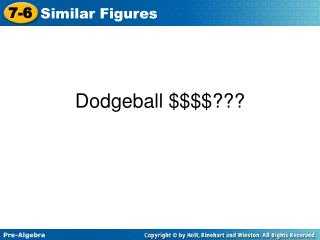DownloadDownload PresentationDodgeball \$\$\$\$???

# Dodgeball \$\$\$\$???

Télécharger la présentation## Dodgeball \$\$\$\$???

- - - - - - - - - - - - - - - - - - - - - - - - - - - E N D - - - - - - - - - - - - - - - - - - - - - - - - - - -
##### Presentation Transcript

1. Dodgeball \$\$\$\$???

2. 7-6 Similar Figures p 9 3 9 y 5 28 26 56 35 4 12 56 m b 30 = = = = Pre-Algebra Warm Up Solve each proportion. 1. 2. b = 10 y = 8 m = 52 3. 4. p = 3

3. Congruent figures have the same size and shape. Similar figures have the same shape, but not necessarily the same size. The ratio formed by the corresponding sides is the scale factor.

4. AB and XY BC and YZ AC and XZ Remember! The following are matching, or corresponding: A and X A X Y Z C B B and Y C and Z

5. Additional Example 3: Identifying Similar Figures Which rectangles are similar? Since the three figures are all rectangles, all the angles are right angles. So the corresponding angles are congruent.

6. Try This: Example 3 Which rectangles are similar? 8 ft A 6 ft B C 5 ft 4 ft 3 ft 2 ft Since the three figures are all rectangles, all the angles are right angles. So the corresponding angles are congruent.

7. Do examples

8. Check homework